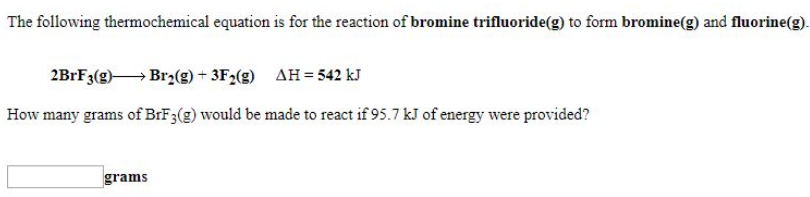# Problem: The following thermochemical equation is for the reaction of bromine trifluoride(g) to form bromine(g) and fluorine(g). 2BrF3 (g) → Br2 (g) + 3F2 (g)       ΔH = 542 kJ How many grams of BrF3 (g) would be made to react if 95.7 kJ of energy were provided?

###### FREE Expert Solution
89% (201 ratings)###### Problem Details

The following thermochemical equation is for the reaction of bromine trifluoride(g) to form bromine(g) and fluorine(g).

2BrF3 (g) → Br2 (g) + 3F2 (g)       ΔH = 542 kJ

How many grams of BrF3 (g) would be made to react if 95.7 kJ of energy were provided?Frequently Asked Questions

What scientific concept do you need to know in order to solve this problem?

Our tutors have indicated that to solve this problem you will need to apply the Thermochemical Equations concept. You can view video lessons to learn Thermochemical Equations. Or if you need more Thermochemical Equations practice, you can also practice Thermochemical Equations practice problems.